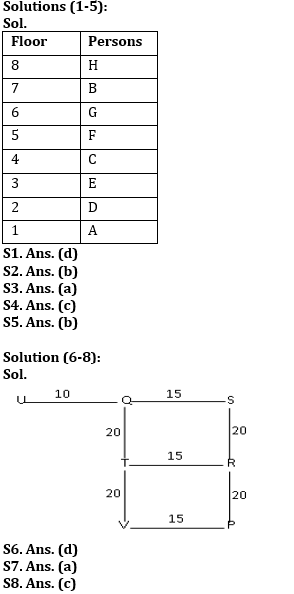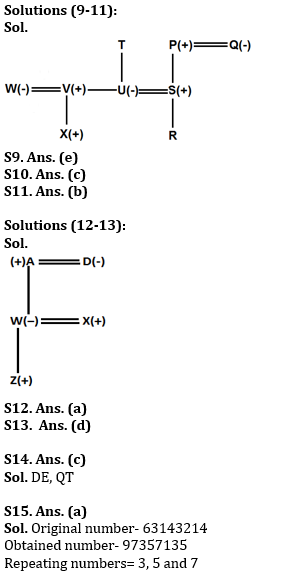Latest Banking jobs   »

# Reasoning Ability Quiz For RBI Grade B/ ECGC PO/ SIDBI Grade A Prelims 2022- 21st April

Directions (1-5): Study the information carefully and answer the question given below.
Eight persons are living on an eight-floor building where ground floor is numbered as 1 and topmost floor is numbered as 8. D lives on an even number floor below 5th floor. There is only one person lives between D and C. G lives on one of the even number floors but not on the top floor. There are two persons living between C and A who lives below E. H lives on a floor immediate above the B’s floor. The total number of persons living between F and B is same as the total number of persons living between F and E.

Q1. Who among the following lives on third floor?
(a) H
(b) G
(c) F
(d) E
(e) None of these

Q2. How many persons live between H and D?
(a) Two
(b) More than Three
(c) Three
(d) One
(e) None

Q3. G lives on which of the following floor?
(a) 6th floor
(b) 4th floor
(c) 2nd floor
(d) 8th floor
(e) None of these

Q4. Who among the following lives immediate below D?
(a) G
(b) F
(c) A
(d) B
(e) None of these

Q5. Which of the following statement is true about F?
(a) E lives one of the floors above F’s floor
(b) Only two floors between F and D
(c) F lives immediate above G
(d) F lives on an even numbered floor
(e) All are true

Directions (6-8): Study the following information carefully and answer the given questions.
Point S is 40 m towards the North of Point P. Point Q is 15 m towards the West of point S. Point U is 25 m towards the West of point S. Point T is 20 m towards the South of point Q. Point R is 15 m towards the East of point T. Point V is 15 m towards the West of point P.

Q6. Which of the following points are in a straight line?
(a) S, T, P
(b) T, V, R
(c) S, Q, V
(d) T, V, Q
(e) U, Q, R

Q7. P is in which direction with respect to R?
(a) South
(b) North
(c) East
(d) West
(e) Cannot be determined

Q8. If a person walks 10 m towards East from point U and then takes a right turn, which of the following points would he reach first?
(a) V
(b) S
(c) T
(d) P
(e) R

Directions (9-11): Study the information carefully and answer the questions given below.
There are nine members in a family in which only three married couples. There are three generation in this family. Q is the wife of P. P is the grandfather of R. Q has only one son who is married to T’s child. T has only two children one son and one daughter. X is grandson of T. S is brother-in-law of son of T. U and V are children of T. W is married to the son of T. X is nephew of U and he is W’s child. U is a married woman.

Q9. If R is married to Y, then how is Y related to S?
(a) Son
(b) Daughter
(c) Son-in-law
(d) Daughter-in-law
(e) Can’t be determined

Q10. How is S related to T?
(a) Son
(b) Daughter
(c) Son-in-law
(d) Daughter-in-law
(e) Husband

Q11. How is Q related to R?
(a) Grandfather
(b) Grandmother
(c) Uncle
(d) Aunt
(e) Either (c) or (d)

Directions (12-13): Study the following information carefully and answer the given questions:
In a family of five members, there are two married couples and three generations in the family. A is father-in-law of X who is married with W. Z is the son of W. D who is a married woman has only one daughter.

Q12. How is A related with Z?
(a) Grand Father
(b) Grand Mother
(c) Father-in-law
(d) Daughter in law
(e) Grandson

Q13. Who is the son-in-law of Z’s grandmother?
(a) A
(b) D
(c) W
(d) X
(e) None of these

Q14. How many pairs of letters are there in the word “ADEQUATE” each of which have as many letters between them in the word as they have between them in the English alphabetical series (both forward and backward direction)?
(a) Three
(b) One
(c) Two
(d) More than three
(e) None

Q15. If in the number 63143214, add 1 after multiplying by 2 in each of the odd digit and subtract 3 after multiplying by 2 in each of the even digit then, how many digits are repeating in new number?
(a) Three
(b) One
(c) None
(d) Two
(e) Four

Solutions